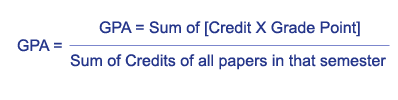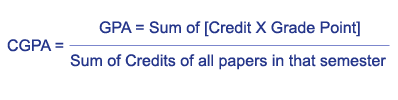# How to calculate CGPA?

How to Calculate CGPA?
CGPA stands for cumulative grade point average. The CGPA is a figure that reflects the grade point average for all classes you have taken and for classes for which you have received credit by means such as testing at your school/College/University.
School/College/university policies vary in the way they evaluate credit for courses transferred to your current school from another. Your university's registrar will have information about your university's policy.
To calculate your CGPA you need to know the total number of grade points you have earned and the total number of credit hours you have attempted.The virtual university CGPA also follows the same pattern of calculation.

## Step 1.

Determine the total number of credit hours you have attempted. For example, after two semesters you might have attempted 24 credit hours. This figure can be found on an official transcript from your school.

## Step 2.

Determine the total number of grade points you have earned. For example, you might have earned 72 grade points in two semesters. As with the number of credit hours attempted, this figure can be found on your school transcript.

## Step 3.

Enter the number of grade points earned and credit hours attempted into the CGPA formula: Grade points earned divided by credit hours attempted equals cumulative grade point average. Using the example figures, this would be: 72 grade points earned divided by 24 credit hours attempted equals cumulative grade point average.

## Step 4.

Apply the CGPA formula using the figures you have entered to calculate your CGPA. With the example figures, the CGPA is 3.0.

## Here we have the formula as well:

CGPA=SUMMATION (summation)
(OF NUMBER OF CREDITS OF THE ith course of the
nth semester and grade points of the ith course of the nth semester) /summation (summation) number of credits of the ith course of the n th semester

## What is GPA?

GPA is the ratio of the sum of the products of the number of credits of courses registered and the grade points corresponding to the grades scored in those courses, taken for all the courses, to the sum of the number of credits of all the courses in the semester.

## GPA calculator:## CGPA calculator:

CGPA will be calculated in a similar manner, considering all the courses enrolled from first semester## Restriction:

The CGPA does not form part of the student record for the Faculty of Medicine and most graduate studies programs.

###### Share To: